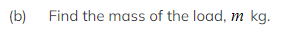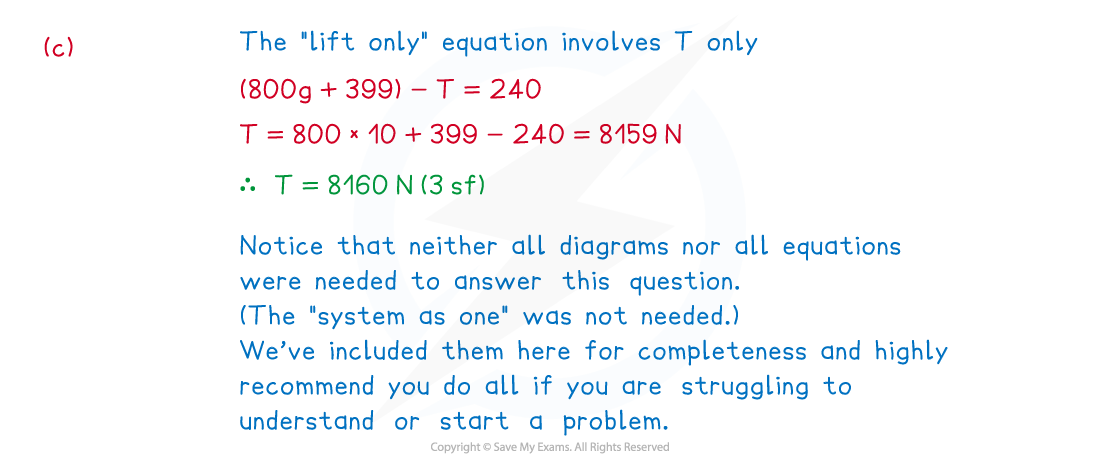# CIE A Level Maths: Mechanics复习笔记3.2.3 Connected Bodies - The Lift Problem

### Connected Bodies - The Lift Problem

#### What is the lift problem?

• The lift problem involves objects (particles) that are directly in contact with each other – typically a person or crate in a lift
• If it is not a person in the lift the object is often referred to as a load
• There may be more than two objects involved – for example two crates stacked on top of each other on a lift floor
• Vertical motion is involved so use g m s-2, the acceleration due to gravity, where appropriate
• Gravity always acts vertically downwards
• Depending on the positive direction chosen – and which other forces are acting vertically – acceleration (a m s-2) may be positive or negative
• Remember that acceleration links F= ma (N2L) and the ‘suvat’ equations

#### How do I solve ‘lift problem’ type questions?

• Lift problems will only consider motion in the vertical direction
• As motion is involved Newton’s Laws of Motion apply so use “F = ma” (N2L)
• The steps for solving lift problems are the same as for solving rope problems
• As both the lift and load are travelling in the same direction the system can be treated as one particle (as well as separate particles)
• There is no reaction force acting on the lift or load when treating the particle as one - mathematically they cancel each other out
• You can think of the upward R N as counteracting the person’s weight and moving the load upwards; N3L applies so there must be an equal force acting in the opposite direction; - you can think of this as the force keeping the person in contact with the lift floor whilst it is moving
• For constant acceleration the ‘suvat’ equations could be involved#### How do we form the equations for problems involving tow bars and ropes?

• Form the equations as follows:
• Treating the lift and person/load as one

(↓) (M + m)g - T = (M + m)a

• Treating the lift and person/load separately

Lift: (↓) (Mg + R) - T = Ma

Person/load: (↓) mg - R = ma

• You do not necessarily need all equations but if in doubt attempt all and it may help you make progress

#### Worked Example(a)Briefly explain how the force of 800g N arises in this problem.(c)Find the tension, T N, in the cable of the lift.#### Exam Tip

• Sketch diagrams or add to any diagrams given in a question.
• If in doubt of how to start a problem, draw all diagrams and try writing an equation for each.  This may help you make progress as well as picking up some marks.
• Watch out for “hidden lift” problems – we’re not strictly talking elevators here!  For example, a load being raised by a crane; the “lift” would be a platform (such as a pallet) and the “lift cable” would be the cable connecting the crane to the load. Another common alternative is a fast rising (or falling) fairground ride.